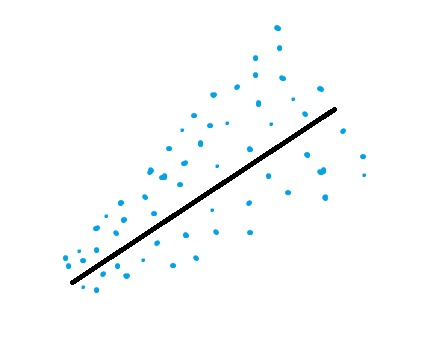# Park Test: Definition, How to Run

Statistics Definitions > Park Test

## What is the Park Test?Heteroscedastic data.The Park Test is a test for heteroscedasticity. Heteroscedasticity means that the variances of the errors are not the same across a set of independent (predictor) variables. Use the Park test for heteroscedasticity if you have some variable Z that you think might explain the different variances of the residuals.

There are different forms of this test: the log form is the most common and is the one outlined here, where: LN(Residual2) = b(intercept) + slope(m) (LN(X)). However, you can theoretically use another form, like the linear form Residual2 = b + m(X). The linear form is the same as the Breusch Pagan test.

To run the test, regress the natural log of squared residuals against the independent variable. If the independent variable has a significant b coefficient, the data is likely heteroscedastic.

## Steps for Running a Park Test

Step 1 : Run ordinary least squares on your data. Make sure the regression produces a table of residuals.

Step 2 : Square the residuals from Step 1.

Step 3 : Take the natural log of the squared residuals from Step 2.

Step 4 : Take the natural log of Z, the variable which you suspect is causing the heteroscedastic behavior.

Step 5 : Run OLS again, this time for the natural log of Z (Step 4) against the natural log of the squared residuals (Step 3). In other words, LN of Z is your independent variable and LN(residuals2) is your dependent variable for the regression.

Step 6 : Find the T-Statistic for the Z variable. A large t-statistic (i.e. over 2) indicates the presence of heteroscedasticity.

Caution: There has been some debate about the validity of this test. For example, Goldfeld and Quandt cautioned that vi may violate assumptions of OLS.

Reference:
Goldfeld, Stephen M.; Quandt, Richard E. (1972) Nonlinear Methods in Econometrics, Amsterdam: North Holland Publishing Company, pp. 93–94. Referred to in: Gujarati, Damodar (1988) Basic Econometrics (2nd Edition), New York: McGraw-Hill,p. 329.

CITE THIS AS:
Stephanie Glen. "Park Test: Definition, How to Run" From StatisticsHowTo.com: Elementary Statistics for the rest of us! https://www.statisticshowto.com/park-test/
---------------------------------------------------------------------------Need help with a homework or test question? With Chegg Study, you can get step-by-step solutions to your questions from an expert in the field. Your first 30 minutes with a Chegg tutor is free!